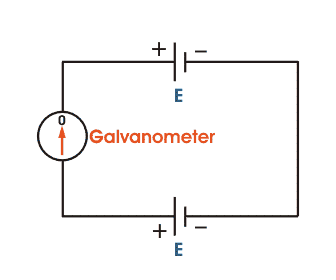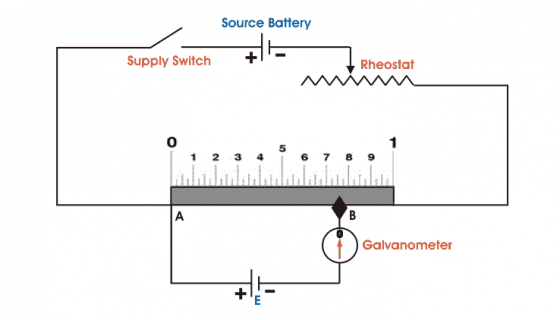×#### Thank you for registering.

One of our academic counsellors will contact you within 1 working day.

Click to Chat

1800-1023-196

+91-120-4616500

CART 0

• 0

MY CART (5)

Use Coupon: CART20 and get 20% off on all online Study Material

ITEM
DETAILS
MRP
DISCOUNT
FINAL PRICE
Total Price: Rs.

There are no items in this cart.
Continue Shopping
```
the emf of a cell used in the main circuit of the potentiometer should me more than the potential difference to be measured . why?
the emf of a cell used in the main circuit of the potentiometer should me more than the potential difference to be measured . why?

```
4 years ago

```							Potentiometer is a very basic instrument used for comparing emf of two cells and for calibrating ammeter, voltmeter and watt-meter. Basic working principle of potentiometer is very simple. If we have connected two batteries head to head and tail to tail through a galvanometer, it means positive terminals of both batteries are connected together and negative terminals are also connected together through a galvanometer as shown in figure below:In the figure, it is clear that if voltage of both battery cells is exactly equal, there will be no circulating current in circuit and hence galvanometer shows null deflection. Working principle of potentiometer depends on this phenomenon.Now, let us think about another circuit, where a battery is connected across a resistor via a switch and a rheostat. There will be a voltage drop across resistor. As there is a voltage drop across resistor, this portion of circuit can be considered as a voltage source for other external circuits. That means anything connected across the resistor will get voltage. If resistor has uniform cross-section throughout its length, electrical resistance per unit length of resistor is also uniform throughout its length. Hence, voltage drop per unit length of the resistor is also uniform. Suppose current through resistor is i amperes & resistance per unit length of resistor is r Ω. Then, voltage appears per unit length across the resistor would be ‘ir’ which is say v volt.Positive terminal of a standard cell is connected to point A on sliding resistor and negative terminal of the same is connected with a galvanometer. Other end of galvanometer is in contact with resistor via a sliding contact in figure above. By adjusting this sliding end, a point like B is found where there is no current through galvanometer, hence there is no deflection of galvanometer. This means emf of standard cell is just balanced by voltage drop across AB. If distance between point A and B is L, then it can be written asEmf of standard cell E = Lv volt.As v (voltage drop per unit length of sliding resistor) is known and L is measured from scale attached to resistor, value of E  or emf of standard cell can be calculated from above simple equation easily.One of the uses of potentiometer is to compare emfs of different cells. Here’s how a DC potentiometer can compare emfs of two different cells. Assume two cells whose emfs are to be compared are joined. Positive terminals of cells and source battery are joined together. Negative terminals of cells are joined with galvanometer in turn through a two-way switch. Other end of galvanometer is connected to sliding contact on the resistor. By adjusting sliding contact on resistor, null deflection of galvanometer comes for first cell at a length of L on scale and after positioning two way switch to second cell and by adjusting sliding contact, null deflection of galvanometer comes for that cell at length of L1 on scale. Think of first cell as standard cell and its emf is E and second cell is unknown cell whose emf is E1.E = Lv volt andL1= L1v voltDividing one equation by other, we get: As the emf of standard cell is known, emf of unknown cell can be determined.Voltmeter measures voltage across terminals of a cell when cell is in closed circuit (when current is flowing through cell). This voltage is not equal to ‘emf’ of cell. It is equal to emf – potential drop across internal resistance of cell. Thus, voltmeter reading V = EMF – i*r, where r is internal resistance.In contrast, voltage measured using potentiometer is voltage across terminals of the cell when current is not flowing through it. (as at null point, there is no current through cell). This voltage is exactly emf of cell.Accuracy of a potentiometer can be increased to a great extent by increasing length of ‘potentiometer wire’. Accuracy of a voltmeter cannot be increased beyond a limit.A potentiometer does not measure voltage although it can produce a potential difference. It’s a resistor with a sliding tap. Resistance between outer terminals has a fixed value and depending on position of tap, a % of that resistance is presented between tap and outer terminals.Potentiometer can measure voltage across entire circuit including that across internal resistance.For a potentiometer:E=I*(R+r)Potentiometer is a simple device used to measure EMF, TPD, internal resistance of a cell. It consists of a board where a tungsten wire is fitted on it. It works on principle that potential dropped between two points in a wire of uniform cross section is directly proportional to distance between the points.Driving cell (E) of some EMF which is always greater than EMF (E’) to be measured is used to send current through circuit. It drops uniform potential along potentiometer wire.Between A and X (some point in between A & B), some potential (V) is dropped. Consider alternative path wire AGX for flow of current in between A and X except potentiometer wire. Potential due to driving cell is same for both AX segment of wire and long wire AGX as they are in parallel. So, electric field exists along AGX. When cell E’ is introduced with positive connected to A in path AGX, it creates opposite field to that created by driving cell and thus when V=E’, no current flows through portion AGX which is confirmed by galvanometer. Thus, we get E’∝ d.
```
4 years ago
Think You Can Provide A Better Answer ?

## Other Related Questions on Electric Current

View all Questions »### Course Features

• 101 Video Lectures
• Revision Notes
• Previous Year Papers
• Mind Map
• Study Planner
• NCERT Solutions
• Discussion Forum
• Test paper with Video Solution### Course Features

• 110 Video Lectures
• Revision Notes
• Test paper with Video Solution
• Mind Map
• Study Planner
• NCERT Solutions
• Discussion Forum
• Previous Year Exam Questions